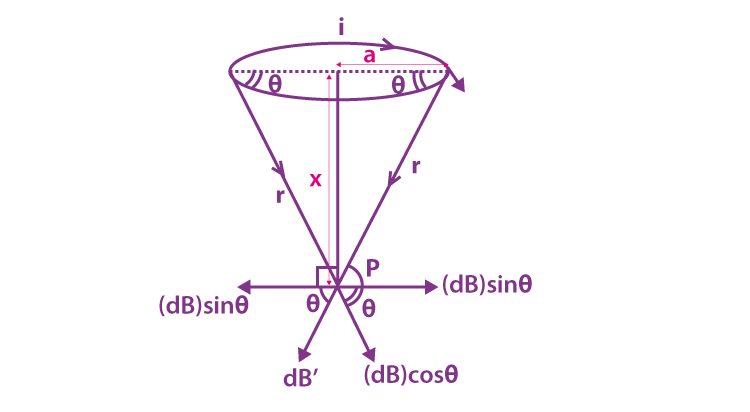# Magnetic Field Intensity In A Circular Loop

## What Is Magnetic Field Intensity?

The magnetic field intensity is defined as the ratio of magnetomotive force that is required to create a flux density within a material per unit length of that material.

The magnetic field intensity is also known as the magnetic field strength. H is the symbol that is used for describing the magnetic field intensity. The SI unit of the magnetic field intensity is Ampere/meter (A/m).

## Magnetic Field Intensity Due To A Circular Loop Of A Current-Carrying Conductor

The magnetic field intensity for a circular loop is considered across its axial position. For this, let “a” be the radius of the circular loop. The current flowing through the circular loop is “i”. The intensity of the magnetic field is determined at the point “P”. This point is at a distance of “x” from the centre of the circular loop.

$$d\vec{B}$$ and $$d\vec{B’}$$ are the two diametrically opposite current lengths and the magnetic field intensity. These two elements are used for determining the intensity of the magnetic field at the point “P”.

The below figure shows the pictorial representation of the circular loop carrying current through it:$$d\vec{B}$$ and $$d\vec{B’}$$ are resolved into two mutually perpendicular components. These mutually perpendicular components are added along the axis because they are in the same direction such that they are perpendicular to the axis. This makes them cancel out each other.

The magnetic field due to current length $$id\vec{l}$$ is given as:

$$d\vec{B}=\frac{\mu _{0}(id\vec{l} \times \vec{r})}{4\pi r^{3}}$$

The magnetic field intensity due to the current-carrying circular loop along the axial position is given as:

$$\vec{B}=\int d\vec{B}$$

Since the axial position is considered, the intensity of the magnetic field will be along the axis.

So,

$$B=\int (dB)cos\theta$$ $$B=\int[\frac{\mu _{0}}{4\pi}\frac{i(dl)sin90^{\circ}}{r^{2}}]\frac{a}{r}$$ $$B=\frac{\mu _{0}}{4\pi}\frac{ia}{r^{3}}\int dl$$

We got the above equation since $$r=\sqrt{a^{2}+x^{2}}$$ $$B=\frac{\mu _{0}}{4\pi }\frac{ia}{(a^{2}+x^{2})^\frac{3}{2}}\int dl$$ $$B=\frac{\mu _{0}}{4\pi }\frac{ia}{(a^{2}+x^{2})^\frac{3}{2}}(2\pi a)$$ $$B=\frac{\mu _{0}ia^{2}}{2(a^{2}+x^{2})^\frac{3}{2}}$$

Therefore, the above equation is the magnetic field intensity of a circular current-carrying loop.

If there are N number of turns in the loop, then the magnetic field is given as:

$$B=\frac{\mu _{0}Nia^{2}}{2(a^{2}+x^{2})^\frac{3}{2}}$$

For a better understanding of the magnetic field and the magnetic field lines, please refer to the below video:## Frequently Asked Questions – FAQs

### The quantity H is analogous to which component?

The quantity H is the magnetic field intensity, and it is analogous to E which is the electric field intensity.

### What is the unit of magnetic field intensity?

The unit of magnetic field intensity is Amperes/meter.

### What is the unit of magnetic flux density?

The unit of magnetic flux density is Weber/m2

### What is the difference between the magnetic field intensity and the magnetic flux density?

The difference between the magnetic field intensity and the magnetic flux density is that the magnetic field intensity is the amount of magnetising force whereas the magnetic flux density is the measure of magnetic force that is induced on a given material due to the magnetising force.

### Can we see magnetic flux lines?

It is not possible to see the magnetic flux lines. However, it can be visualized with the help of the iron fillings. When the iron fillings are sprinkled on a sheet of paper, they get attracted to the magnet. At the poles we can notice that the iron fillings are dense while on the other parts of the magnet, the iron fillings are not that dense. This shows the lines of force on the magnet which is the magnetic flux.